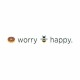Isha Arora — October 12, 2020

This article was published as a part of the Data Science Blogathon.

## Introduction

I have always been in love with Data Visualization since the day I started working on it. I always enjoy deriving useful insights from the data. Before this, I only knew about basic charts like bar graphs, scatter plots, histograms, etc those are inbuilt in tableau and power BI in data visualization. By working every day on this task, I came across many new charts like radial gauge chart, waffle charts, and so on.

So, out of my curiosity, recently I was searching for all the types of charts that are used in data visualization, where this word cloud caught my eye and I found it very interesting. Till now, seeing these word cloud images forced me to think that these are just random images where those words are randomly arranged, But I was wrong, and where it all started. After this, I tried making word cloud from just small data in Tableau and Power BI. After that successful attempt, I wanted to try it by code alike making bar charts, pie charts, and other charts.

## What basically A Word Cloud is?

Definition: A word cloud is a simple yet powerful visual representation object for text processing, which shows the most frequent word with bigger and bolder letters, and with different colors. The smaller the the size of the word the lesser it’s important.

## Uses of Tag Cloud

1) Top Hashtags on Social Media(Instagram, Twitter): Throughout the world, social media is trending for the latest updates, so from that we can get the most used Hashtags that people use in their posts.

2) Hot Topics In Media: Analyzing the news articles, we can find the keywords in the headlines and extract the top n demanding topics and to get the desired result i.e the top n trending media topics.

3)Search Term in an E-commerce: In an e-commerce shopping website, the owner can make the word cloud of the shopping items that have been searched the most. So, he can get the idea about which shopping is in great demand during a specific period.

## Let’s Start Coding in python to achieve this kind of word cloud

First of all, we need to install all the libraries in the jupyter notebook.

So, in python, there is an inbuild library wordcloud which we will install. In the Anaconda Command prompt write the following code:

`pip install wordcloud`

If your anaconda environment supports conda, then write:

`conda install wordcloud`

Although, this can directly be achieved in the notebook itself, just by adding ‘!’ at the beginning of the code

Like:

`!pip install wordcloud`

Now, here I will generate the wordcloud of the Wikipedia text of any topic. So I will need a Wikipedia library to access Wikipedia API which can be done by installing Wikipedia  in anaconda command prompt as follow:

`pip install wikipedia`

Now there are some other libraries which we are needed, they are numpy. matplotlib and pandas.

As of now, we have all the libraries to create the tag cloud

`import wikipedia`
```result= wikipedia.page("MachineLearning")
final_result = result.content
print(final_result)```

The above is the image of the output that we got by retrieving the machine learning page of Wikipedia. There we will also be able to see the scroll down, which means the entire page is retrieved.

Here, we can also get the summary of the page by the summary method as below: and

```result= wikipedia.summary("MachineLearning", sentences=5)
print(result)```

Here we have the parameter of sentences, so we can use it to retrieve a specific number of lines.

The output of 5 sentences

#### Let’s have the wordcloud now

```from wordcloud import WordCloud, StopWords
import matplotlib.pyplot as plt
def plot_cloud(wordcloud):
plt.figure(figsize=(10, 10))
plt.imshow(wordcloud)
plt.axis("off");
wordcloud = WordCloud(width = 500, height = 500, background_color='pink', random_state=10).generate(final_result)
plot_cloud(wordcloud)```

Stopwords are the words which does not have any meaning like ‘is’, ‘are’, ‘an’, ‘I’ and many more.

Wordcloud comes with an inbuilt library of stop words, that will automatically remove the stop words from the text.

But, an interesting thing that comes here is that we can add our choice of stop words in python by stopwords.add() function.

Wordcloud method will have width and height to set, I have set both of them as 500, background color as pink.  If you do not add a random state, then every time you run your code, your word cloud will look different. It should be set as an int value.

Here is the desired word cloud, we will get from the above code:

By seeing the above figure, we see that machine learning is the most used word, and there are some other words that are frequently used are model, task, training, data. So we can conclude that machine learning is the task of training the data model.

We can also change the background color by background color method and the font colors by colormap method here and we can also add the hash codes of the colors in background color, but the mapcolor comes with the inbuild specific colors.

Let’s change the background color to turquoise by using its hash code and font colors to blue:

```from wordcloud import WordCloud, StopWords
import matplotlib.pyplot as plt
def plot_cloud(wordcloud):
plt.figure(figsize=(10, 10))
plt.imshow(wordcloud)
plt.axis("off");
wordcloud = WordCloud(width = 500, height = 500, background_color='#40E0D0', colormap="ocean",  random_state=10).generate(final_result)
plot_cloud(wordcloud)```

Here, I have specified ocean, if I add some wrong color map, jupyter will throw a value error and show me the available options for color map as below:

Wor cloud can also be implemented in any image by using the PIL library.

## End Notes

In this article, we discussed about word cloud, its definition, its application areas, and its example in python using jupyter notebook.###### Isha Arora